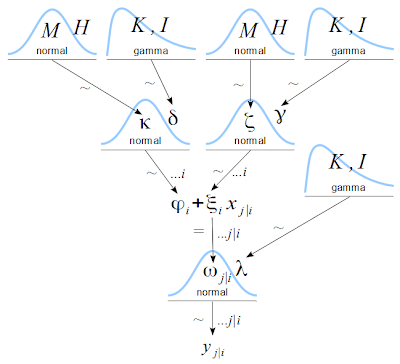## Thursday, October 31, 2013

### Diagrams for hierarchical models: New drawing tools

Two new drawing tools for making hierarchical diagrams have been recently developed. One tool is a set of distribution and connector templates in LibreOffice Draw and R, created by Rasmus Bååth. Another tool is scripts for making the drawings in LaTeX via TikZ, created by Tinu Schneider. Here is an example of a diagram made by Tinu Schneider, using TikZ/LaTeX with Rasmus Bååth's distribution icons:
The drawing language TikZ is very powerful and can create very elaborate illustrations. Here is a variation in which the distribution nodes have boxes around them:

The distribution icons above are images created in R with a program by Rasmus Bååth. The program generates a catalog of icons with the same visual formatting. The icons can then be used in TikZ/LaTeX as shown in Tinu Schneider's drawings above ...

... or they can be used with freehand drawing in LibreOffice Draw. Rasmus has created a screencast that shows how do use the icons in LibreOffice Draw:

If you use LaTeX and you want precise positioning of nodes, ability to show wavy arrows and double-line arrows, and math fonts in the diagram that exactly match the math fonts in the text, then the TikZ/LaTeX tools are for you. If you want WYSIWYG editing, then LibreOffice is for you.

Rasmus Bååth's blog post about his system is here. In particular, the LibreOffice template is here, and the R code for generating the distributions is at his Github folder. You can download LibreOffice here. (You might recall that Rasmus also created the in-browser app for "Bayesian estimation supersedes the t test" (BEST) available here.)

Tinu Schneider's description of his TikZ/LaTeX examples are in this README document at his Github folder. Info about the TikZ package is here, and it can be downloaded here. (You might recall that Tinu also created the dynamic visualization of the Metropolis algorithm with rejected proposals at this blog post.)

Big THANKS to Rasmus and Tinu for creating these terrific tools!

## Wednesday, October 9, 2013

### Diagrams for hierarchical models - we need your opinion

When trying to understand a hierarchical model, I find it helpful to make a diagram of the dependencies between variables. But I have found the traditional directed acyclic graphs (DAGs) to be incomplete at best and downright confusing at worst. Therefore I created differently styled diagrams for Doing Bayesian Data Analysis (DBDA). I have found them to be very useful for explaining models, inventing models, and programming models. But my idiosyncratic impression might be only that, and I would like your insights about the pros and cons of the two styles of diagrams.

To make the contrast concrete, let's consider the classic "rats" example from BUGS. I will start with a textual explanation of the model (using modified phrasing and variable names), and then provide two diagrams of the model, one in DAG style and one in DBDA style. Here is the text:
The data come from 30 young rats whose weights were measured weekly starting at birth for five weeks, with the goal being to assess how their weights changed as a function of age. The variable yj|i denotes the weight of the ith rat measured at days since birth xj|i. The weights are assumed to be distributed normally around the predicted value ωj|i (omega):
yj|i ~ normal( ωj|i , λ )
The parameter λ (lambda) represents the precision (i.e., 1/variance) of the normal distribution. The predicted weight of the ith rat is modeled as a linear function of days since birth:
ωj|i = φi + ξi xj|i
The individual intercepts φi (phi) and slopes ξi (xi) are assumed to come from group-level normal distributions,
φi ~ normal( κ , δ )    and    ξi ~ normal( ζ , γ )
where κ (kappa) and ζ (zeta) are the means of the group-level distributions. The priors on the group-level means are set as vague normal distributions,
κ ~ normal( M , H )    and    ζ ~ normal( M , H )
where the mean M is approximately central on the scale of the data and the precision H is very small. The precision parameters, λ (lambda), δ (delta), and γ (gamma), are given vague gamma priors,
λ ~ gamma( K , I )    and    δ ~ gamma( K , I )    and    γ ~ gamma( K , I )
where the shape K and rate I parameters are set to very small values.
Below are two different diagrams of the model. The first is a DAG. Because there are a variety of style conventions in the literature for DAGs, I used my own hybrid explained in the caption. But I think it is a good and useful hybrid, one that I would want to use if I used DAGs. Take a look at the DAG:Squares denote constants, circles denote variables. Solid arrows denote stochastic dependency, heavy dotted arrows denote deterministic dependency. Rounded-corner rectangles denote "plates" for indices.
For DAG users, does the style above capture what you think is needed in a DAG? Does the DAG above help you understand the model? How? Does the DAG confuse you? Why? Would the DAG help you program the model in BUGS / JAGS / Stan? Or not?

Below is a DBDA-style diagram for the model:Arrows marked by "~" denote stochastic dependency, arrows marked by "=" denote deterministic dependency. Ellipses on arrows denote indices over which the dependency applies.
Does the DBDA-style diagarm above help you understand the model? How? Does the DBDA-style confuse you? Why? Would the DBDA-style help you program the model in BUGS / JAGS / Stan? Or not?